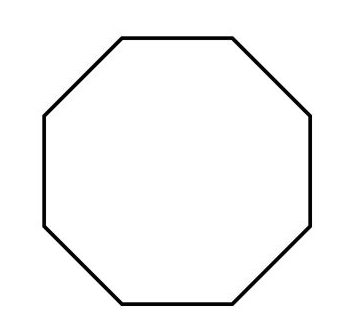Home/Class 8/Maths/

How many sides does an octagon have?（ ）
A. $$7$$
B. $$8$$
C. $$9$$
D. $$10$$Speed
00:00
01:57## QuestionMathsClass 8

How many sides does an octagon have?（ ）
A. $$7$$
B. $$8$$
C. $$9$$
D. $$10$$

B
4.64.6## Solution

Octagon is a $$8$$ sided polygon, as shown in figure below.